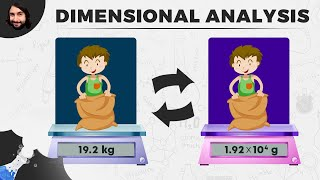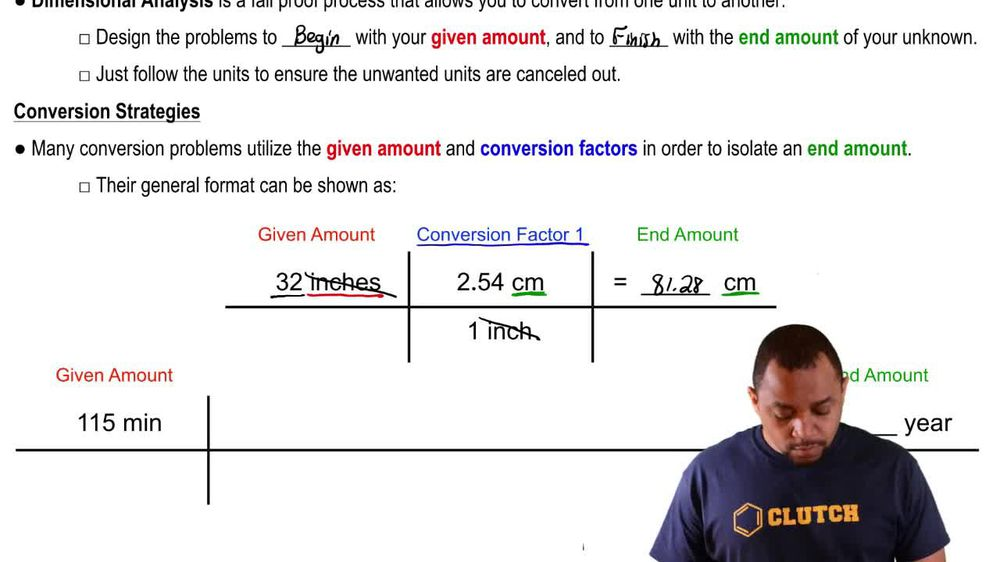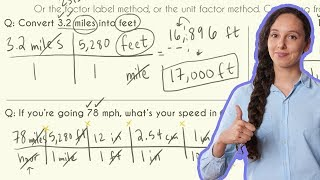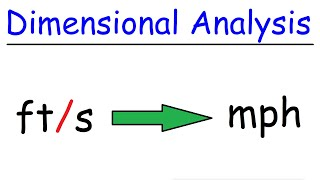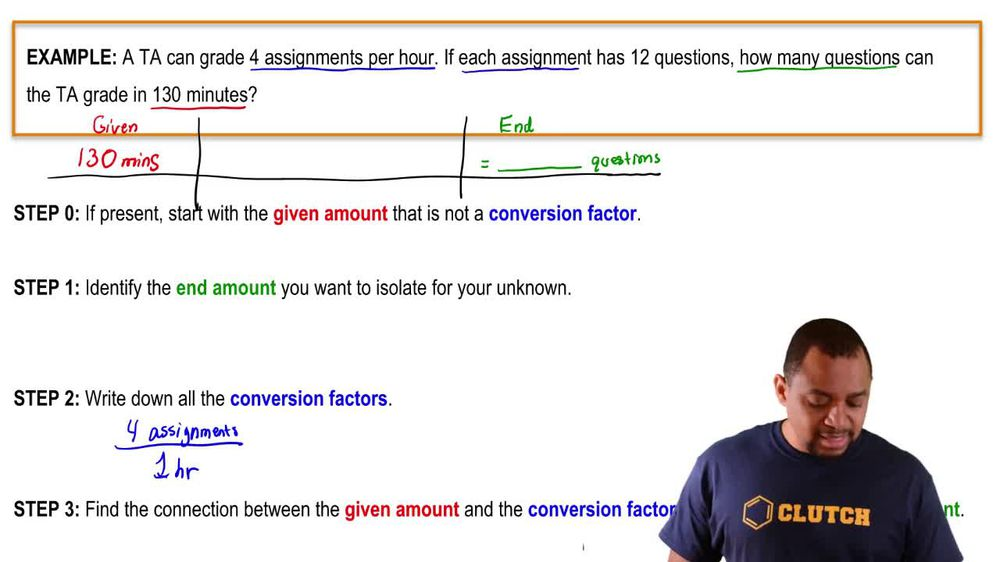Start typing, then use the up and down arrows to select an option from the list.
1. 1. Intro to General Chemistry2. Dimensional Analysis
Problem

# An ibuprofen suspension for infants contains 100 mg>5.0 mL suspension. The recommended dose is 10 mg>kg body weight. How many mL of this suspension should be given to an infant weighing 18 lb? (Assume two significant figures.)

Relevant Solution2m
Play a video:
Hey everyone. So here it says, the recommended acetaminophen suspension dosage for infants is mg per kilogram of body weight. Now, if an acetaminophen suspension contains 70 mg per 700. mL, how much should be given to a £12 infant. Here, we assume the answer to two significant figures. And here all of our answers are in milliliters. Now, if we take a look at the numbers given to us, we have what, 14 mg per one kg, 70 mg per 700.67 millimeters. And then finally £12. Remember always best to start out with the number that has only one unit attached to it. So we're gonna start out with £12. And that's because it's easier to manipulate it if necessary. Now, since I'm starting out with pounds, I need to find where else I can find pounds or or mass. Well here we know that pounds are connected to kilograms. These pounds of the infant can be connected to the kilograms of body weight because they're both dealing with weight. So for me to use these pounds, I'm gonna have to convert them into kilograms. So they can cancel out with these kilograms here. Now, remember the conversion factor is that one kg? It weighs approximately 2.204, 6 lb pounds, cancel out. And now I have kilograms. And now I can bring in my conversion factor here, 14 mg per one kg of body weight. So now I have the milligrams of the suspension and I need to cancel out those milligrams and isolate mls at the end is my answer. So we use this final conversion factor here, Put the 70 mg on the bottom so that the milligrams can cancel out. And then we have the .67 ml on top Here. When everything cancels out, I get as my final answer, .73 mm. This gives me option D as the correct answer.